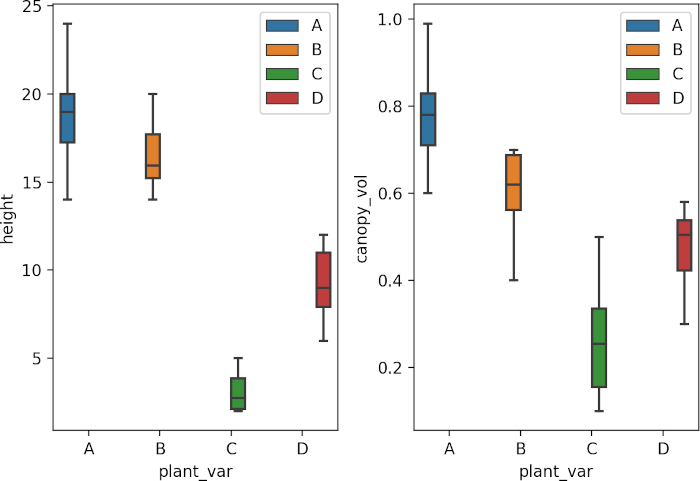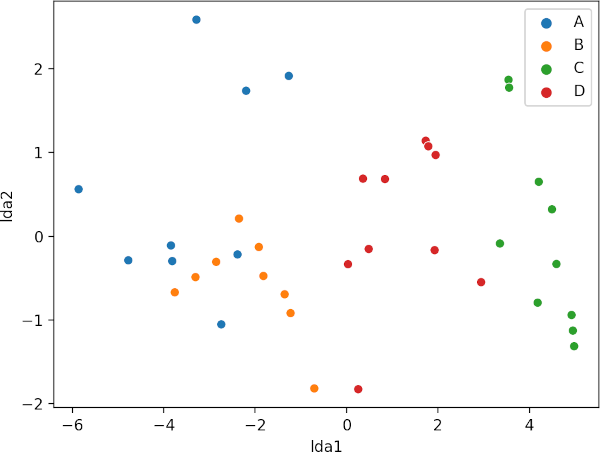# MANOVA using Python (using statsmodels and sklearn)

This article explains how to perform the one-way MANOVA in Python. You can refer to this article to know more about MANOVA, when to use MANOVA, assumptions, and how to interpret the MANOVA results.

## One-way (one factor) MANOVA in Python

### MANOVA example dataset

Suppose we have a dataset of various plant varieties (`plant_var`) and their associated phenotypic measurements for plant heights (`height`) and canopy volume (`canopy_vol`). We want to see if plant heights and canopy volume are associated with different plant varieties using MANOVA.

``````import pandas as pd
plant_var  height  canopy_vol
0         A    20.0         0.7
1         A    22.0         0.8
``````

### Summary statistics and visualization of dataset

Get summary statistics based on each dependent variable,

``````from dfply import *
# summary statistics for dependent variable height
df >> group_by(X.plant_var) >> summarize(n=X['height'].count(), mean=X['height'].mean(), std=X['height'].std())
# output
plant_var   n   mean       std
0         A  10  18.90  2.923088
1         B  10  16.54  1.920185
2         C  10   3.05  1.039498
3         D  10   9.35  2.106735

# summary statistics for dependent variable canopy_vol
df >> group_by(X.plant_var) >> summarize(n=X['canopy_vol'].count(), mean=X['canopy_vol'].mean(), std=X['canopy_vol'].std())
# output
plant_var   n   mean       std
0         A  10  0.784  0.121308
1         B  10  0.608  0.096816
2         C  10  0.272  0.143279
3         D  10  0.474  0.094540
``````

Visualize dataset,

``````import seaborn as sns
import matplotlib.pyplot as plt
fig, axs = plt.subplots(ncols=2)
sns.boxplot(data=df, x="plant_var", y="height", hue=df.plant_var.tolist(), ax=axs)
sns.boxplot(data=df, x="plant_var", y="canopy_vol", hue=df.plant_var.tolist(), ax=axs)
plt.show()
``````### perform one-way MANOVA

``````from statsmodels.multivariate.manova import MANOVA
fit = MANOVA.from_formula('height + canopy_vol ~ plant_var', data=df)
print(fit.mv_test())
# output
Multivariate linear model
==============================================================

--------------------------------------------------------------
Intercept         Value  Num DF  Den DF F Value  Pr > F
--------------------------------------------------------------
Wilks' lambda  0.0374 2.0000 35.0000 450.0766 0.0000
Pillai's trace  0.9626 2.0000 35.0000 450.0766 0.0000
Hotelling-Lawley trace 25.7187 2.0000 35.0000 450.0766 0.0000
Roy's greatest root 25.7187 2.0000 35.0000 450.0766 0.0000
--------------------------------------------------------------

--------------------------------------------------------------
plant_var         Value  Num DF  Den DF F Value  Pr > F
--------------------------------------------------------------
Wilks' lambda  0.0797 6.0000 70.0000  29.6513 0.0000
Pillai's trace  1.0365 6.0000 72.0000  12.9093 0.0000
Hotelling-Lawley trace 10.0847 6.0000 44.9320  58.0496 0.0000
Roy's greatest root  9.9380 3.0000 36.0000 119.2558 0.0000
==============================================================
``````

The Pillai’s Trace test statistics is statistically significant [Pillai’s Trace = 1.03, F(6, 72) = 12.90, p < 0.001] and indicates that plant varieties has a statistically significant association with both combined plant height and canopy volume.

## post-hoc test

Here we will perform the linear discriminant analysis (LDA) using `sklearn` to see the differences between each group. LDA will discriminate the groups using information from both the dependent variables.

``````from sklearn.discriminant_analysis import LinearDiscriminantAnalysis as lda
X = df[["height", "canopy_vol"]]
y = df["plant_var"]
post_hoc = lda().fit(X=X, y=y)

# get Prior probabilities of groups:
post_hoc.priors_
array([0.25, 0.25, 0.25, 0.25])

# get group means
post_hoc.means_
array([[18.9  ,  0.784],
[16.54 ,  0.608],
[ 3.05 ,  0.272],
[ 9.35 ,  0.474]])

# get Coefficients of linear discriminants
post_hoc.scalings_
array([[-0.43883736, -0.2751091 ],
[-1.39491582,  9.32562799]])

# get Proportion of trace (variance explained by each of the selected components)
post_hoc.explained_variance_ratio_
array([0.98545382, 0.01454618])

# plot
X_new = pd.DataFrame(lda().fit(X=X, y=y).transform(X), columns=["lda1", "lda2"])
X_new["plant_var"] = df["plant_var"]
sns.scatterplot(data=X_new, x="lda1", y="lda2", hue=df.plant_var.tolist())
plt.show()
``````The LDA scatter plot discriminates against multiple plant varieties based on the two dependent variables. The C and D plant variety has a significant difference (well separated) as compared to A and B. A and B plant varieties are more similar to each other. Overall, LDA discriminated between multiple plant varieties.